UIHistories Project: A History of the University of Illinois by Kalev Leetaru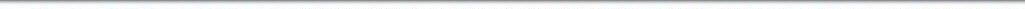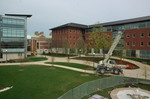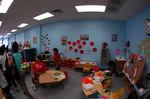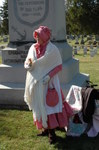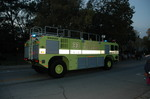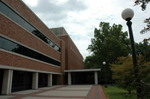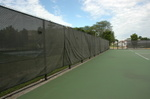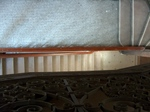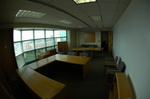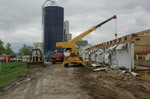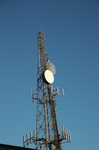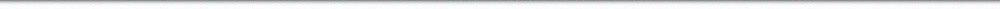N A V I G A T I O N D I G I T A L L I B R A R Y

## Repository: UIHistories Project: Board of Trustees Minutes - 1896 [PAGE 54]

Caption: Board of Trustees Minutes - 1896
This is a reduced-resolution page image for fast online browsing.

< Previous Page [Displaying Page 54 of 371] Next Page >
[VIEW ALL PAGE THUMBNAILS]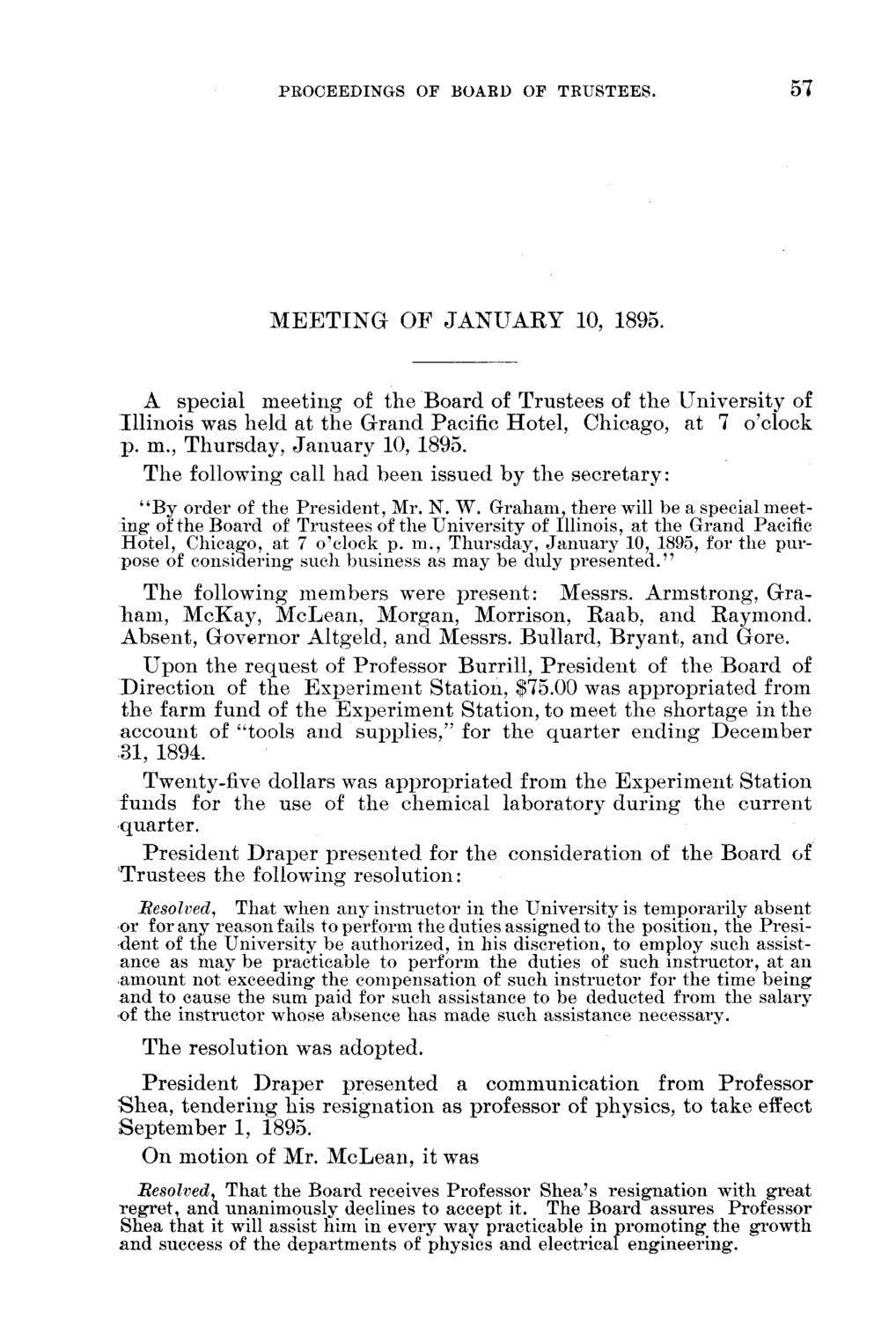### EXTRACTED TEXT FROM PAGE:

PEOOEEDINGS OF BOAED OF TRUSTEES.

57

M E E T I N G O F J A N U A R Y 10, 1895.

A special m e e t i n g of t h e B o a r d of T r u s t e e s of t h e U n i v e r s i t y of I l l i n o i s was held at t h e G r a n d Pacific H o t e l , Chicago, at 7 o'clock p . m., T h u r s d a y , J a n u a r y 10, 1895. T h e following call h a d b e e n issued b y t h e s e c r e t a r y : "By order of the President, Mr. N. W. Graham, there will be a special meeting of the Board of Trustees of the University of Illinois, at the Grand Pacific Hotel, Chicago, at 7 o'clock p. m., Thursday, January 10, 1895, for the purpose of considering* such business as may be duly presented." T h e following m e m b e r s were p r e s e n t : Messrs. A r m s t r o n g , G r a h a m , M c K a y , M c L e a n , M o r g a n , Morrison, R a a b , a n d R a y m o n d . A b s e n t , G o v e r n o r Altgeld, a n d Messrs. B u l l a r d , B r y a n t , a n d Gore. U p o n t h e r e q u e s t of Professor B u r r i l l , P r e s i d e n t of t h e B o a r d of D i r e c t i o n of t h e E x p e r i m e n t S t a t i o n , \$75.00 was a p p r o p r i a t e d from t h e farm fund of t h e E x p e r i m e n t S t a t i o n , to meet t h e s h o r t a g e in t h e a c c o u n t of "tools a n d s u p p l i e s , " for t h e q u a r t e r e n d i n g D e c e m b e r 31, 1894. Twenty-five dollars was a p p r o p r i a t e d from t h e E x p e r i m e n t S t a t i o n f u n d s for t h e use of t h e c h e m i c a l l a b o r a t o r y d u r i n g t h e c u r r e n t quarter. P r e s i d e n t D r a p e r p r e s e n t e d for t h e consideration of t h e B o a r d of T r u s t e e s t h e following r e s o l u t i o n : Resolved, That when any instructor in the University is temporarily absent or for any reason fails to perform the duties assigned to the position, the President of the University be authorized, in his discretion, to employ such assistance as m a y b e practicable to perform the duties of such instructor, at an amount not exceeding* the compensation of such instructor for the time being and to cause the sum paid for such assistance to be deducted from the salary of the instructor whose absence has made such assistance necessary. T h e resolution was adopted. P r e s i d e n t D r a p e r p r e s e n t e d a c o m m u n i c a t i o n from Professor S h e a , t e n d e r i n g h i s r e s i g n a t i o n as professor of physics, to t a k e effect S e p t e m b e r 1, 1895. O n motion of Mr. M c L e a n , it was Besolved, That the Board receives Professor Shea's resignation with great regret, and unanimously declines to accept it. The Board assures Professor Shea that it will assist him in every way practicable in promoting the growth and success of the departments of physics and electrical engineering.